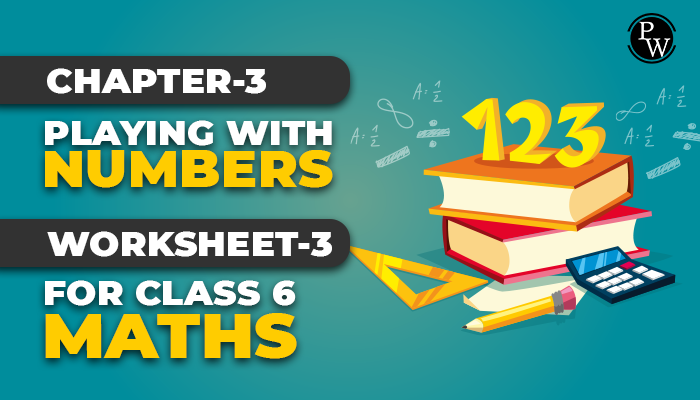# Chapter-3 Playing with Numbers Worksheet-3 for class 6 maths

#### Worksheets for Class 6 Maths

The academic team of Physics Wallah prepared worksheets and questions for the chapter Playing with Numbers for all students who are in class 6. Physics Wallah questions for class 6 maths chapter Playing with Numbers consist of carefully selected numerical for class 6 maths and consist of step-by-step solutions of all questions with answers.

Download worksheet-3 of class 6 maths chapter Playing with Numbers and start solving the questions for reference use the solutions . Before solving the worksheet of Physics Wallah it is highly recommend reading the theory of chapter and solve the questions given in your textbook . Do follow NCERT Solutions for Class 6 Maths prepared by expert faculty of Physics Wallah.For additional information related to the subject you can check the Maths Formula section.

## Solved Examples

Q1. Factors of 34 are 1,2,17,34. Statement which suits above situation is

1. A number can have only four factors
2. Every factor is equal to given number
3. Every factor is less than or equal to given number
4. Every factor is make than the given number

Ans. Correct answer is 3

Explanation: Factor of number is an exact divisor of that number. Take factors of any number say 15, they will be 1,3,5,15.

So, conclusion is “Every factor is less than or equal to given number”.

Q2. “Every multiple of number is greater than or equal to that number”. Option which suits the above condition is

1. Number of multiples of a given number is infinite
2. Multiples of 7 are 7,14,21,28…
3. Factors of 7 are 1 and 7
4. Every number is multiple of itself

Ans. Correct answer is 2

Explanation: Suppose we take multiples of 3 They are 3,6,9,12, so on

So statement “Every multiple of number is greater than or equal to that number” gets justified.

Q3. The correct option is

1. Factors are infinite
2. Multiple are finite
3. 1 is a factor of every number
4. Every number is not a multiple of itself

Ans. Correct answer is 3

Explanation: Take factors of any number say 7 : Factors will be 1,7

9 : Factors will be 1,3,9

So it is true that 1 is a factor of every number.

Q4. The number of multiples of 50 is

1. Limit less
2. 200
3. 150
4. 100

Ans. Correct answer is 1

Explanation: Multiples of any numbers are limitless Example: Multiples of 9 are 9,18,27,36,…………..

Since counting is endless, so are multiples.

Q5. The largest factor of 10576 is

1. 1322
2. 2644
3. 5288
4. 10576

Ans. Correct answer is 4

Explanation: Factors 10576 will be 1,2,4,8,16, 10576

So largest factor will be 10576.

Q6. “1 has only factor 1 itself”. The option which best suits the above condition is

1. 1 is a factor of every number
2. Every composite number has 1 as a factor
3. 1 is a natural number
4. 1 is neither prime nor composite

Ans. Correct answer is 4

Explanation: To become a prime numbers there should be two factors, 1 and the number itself. Also to become a composite number, the number should have atleast three factors. But 1 has only one factor, 1 itself. So 1 is neither prime nor composite.

Q7. Untrue statement about the number ‘2’ is

1. 2 is smallest prime number
2. Every prime number other than 2 is odd
3. Multiples of 2 are prime numbers
4. 2 is a smallest even number

Ans. Correct answer is 3

Explanation: Take the multiples of 2 They are 2,4,6,8,10,………..

So multiples of 2 are even numbers. So option (c) “ Multiples of 2 are prime numbers” turn out to be false.

Q8. Five consecutive composite numbers between 25 and 50. so that there is no prime number between them are

1. 25,26,27,28,29
2. 32,33,34,35,36
3. 37,38,39,40,41
4. 48,49,50,51,52

Ans. Correct answer is 2

Explanation: Composite numbers are those which have at least three factors

Prime numbers are those which have only two factors 1 and the number itself. So numbers 32,33,34,35,36 are each composite and no prime number between them.

Q9. Number 10824 is divisible by

1. Divisible by 11.
2. Divisible by 3,11.
3. Divisible by 2,3,4,6,9,11
4. Divisible by 2,3,4,6,11

Ans. Correct answer is 4

Explanation: For divisible by 2 number should have 0,2,4,6,8 in its unit place. 10824 has 4 in its unit place so it is divisible by 2

Sum of digits of 10824 is 15,15 is divisible by 3, so 10824 is divisible by 3 Last two digits are 24, so it divisible by 4

Since it is divisible by both 2 and 3, so it divisible by 6

Difference so sum of digits in odd place and sum of digits at even place (from right) is 11, so 10824 is divisible by 11.

Q10. 1023 and 1078 both are divisible by 11. Therefore 1023 + 1078 will be divisible by

1. 3
2. 7
3. 11
4. 13

Ans. Correct answer is 3

Explanation: If two given numbers are divisible by a number, then their sum is also divisible by that number.

### Also Check

Below PDF consist of class 6 maths chapter 3 Playing with Numbers